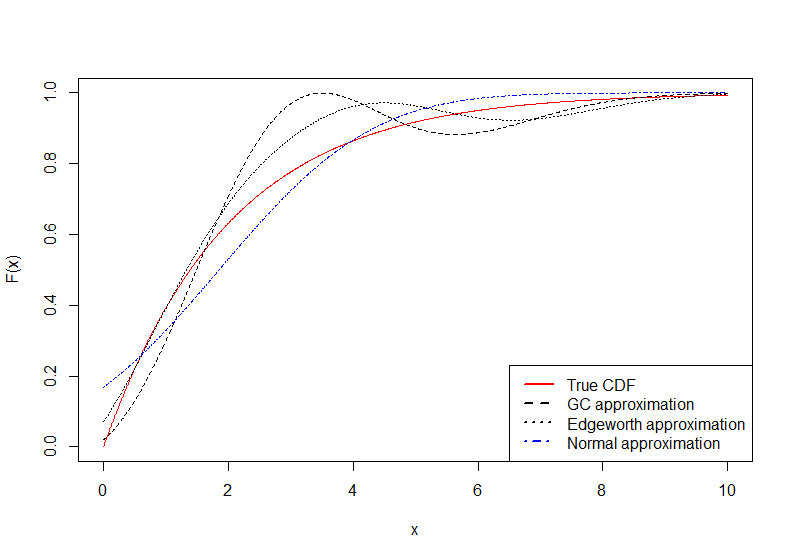# Introduction

The ReIns package contains functions from the book “Reinsurance: Actuarial and Statistical Aspects” (2017) by Hansjörg Albrecher, Jan Beirlant and Jef Teugels.

It contains implementations of

• Basic extreme value theory (EVT) estimators and graphical methods as described in “Statistics of Extremes: Theory and Applications” (2004) of Jan Beirlant, Yuri Goegebeur, Johan Segers and Jef Teugels.

• EVT estimators and graphical methods adapted for censored and/or truncated data.

• Splicing of mixed Erlang distributions with EVT distributions (Pareto, GPD).

• Value-at-Risk (VaR), Conditional Tail Expectation (CTE) and excess-loss premium estimates.

This vignette describes how to use the most important functions of the package. We split this into several sections: datasets, graphical methods, estimators of the extreme value index, estimators of quantiles and return periods, censored data, global fits using splicing, risk measures, distributions and approximations of the distribution function.

# Datasets

Three datasets are available: Norwegian fire insurance data (norwegianfire), SOA group medical insurance data (soa) and Secura Re automobile reinsurance data (secura). These datasets were already discussed in Beirlant et al. (2004).

The illustrations will be done using the Norwegian Fire insurance dataset which contains fire insurance claims for a Norwegian insurance company for the period 1972 to 1992. The sizes of the fire insurance claims are expressed in 1000 NOK.

library("ReIns")

data("norwegianfire")

# Claim year
year <- norwegianfire$year + 1900 # Claim size size <- norwegianfire$size

# Plot Norwegian fire insurance data per year
plot(year, size, xlab="Year", ylab="Size")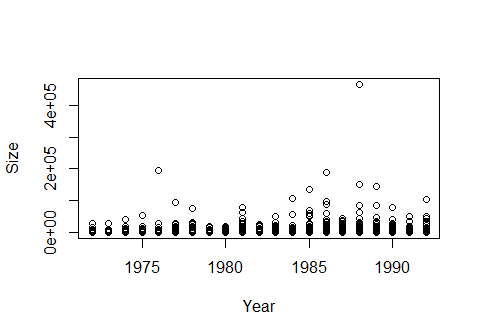# Graphical methods

QQ-plots and their derivative plots are an essential part of extreme value theory. We focus on four important types: the exponential QQ-plot, Pareto QQ-plot, log-normal QQ-plot and Weibull QQ-plot, see Section 4.1 in Albrecher et al. (2017).

## Exponential QQ-plot

The exponential QQ-plot can be easily drawn using ExpQQ. Its derivative plot, also dubbed mean excess plot, is key in determining what type of distribution the data comes from. The mean excess values $$e_{k,n}$$ are plotted using MeanExcess and one has the choice to plot them versus the order statistics $$X_{n-k,n}$$ (k=FALSE) or versus the number of exceedances $$k$$ (k=TRUE).

In this case we see that the mean excess plot is more or less linearly increasing as a function of $$X_{n-k,n}$$ which indicates that the data may come from a Pareto distribution. The exponential QQ-plot is not linear at all but concave which reinforces the conclusion drawn from the mean excess plot.

# Exponential QQ-plot
ExpQQ(size)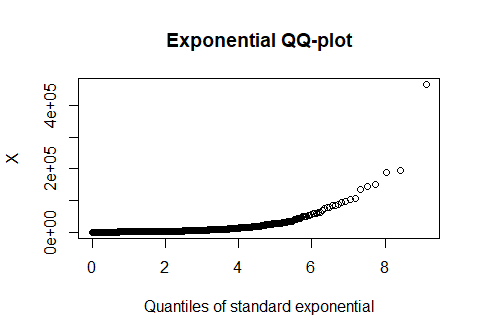# Mean excess plot with X_{n-k,n}
MeanExcess(size)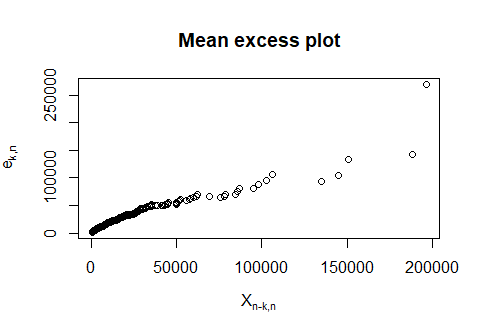# Mean excess plot with k
MeanExcess(size, k=TRUE)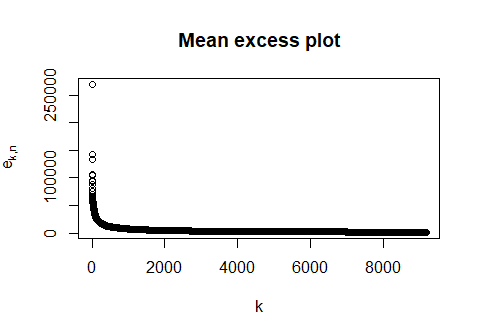## Pareto QQ-plot

Taking the logarithm of Pareto distributed data gives exponentially distributed data, so the Pareto QQ-plot and the exponential QQ-plot are closely related. Using the commands ParetoQQ and ParetoQQ_der, the Pareto QQ-plot and its derivative plot can be drawn. Note that these derivatives are nothing more than the Hill estimates.

The Pareto QQ-plot is now linear which indicates that the Pareto distribution is suitable. The derivative plots can be used to estimate the tail index $$\gamma=1/\alpha$$ of the Pareto distribution (cf. Hill estimator).

# Pareto QQ-plot
ParetoQQ(size)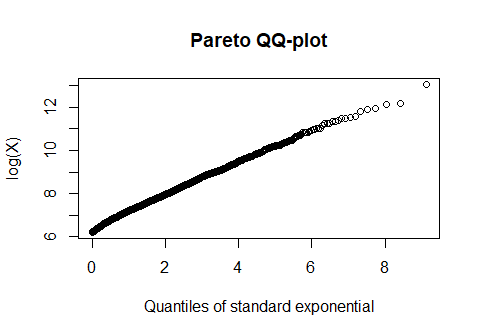# Derivative plot with log X_{n-k,n}
ParetoQQ_der(size)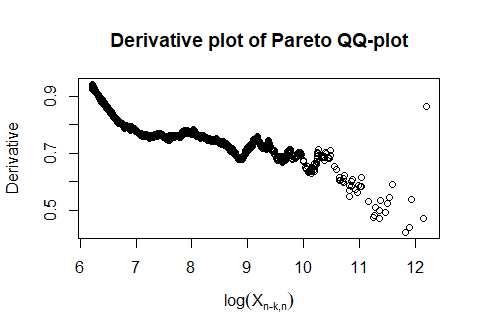# Derivative plot with k
ParetoQQ_der(size, k=TRUE)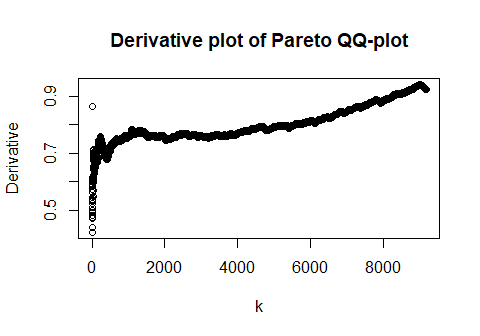## Log-normal QQ-plot

One can also consider the log-normal QQ-plot (LognormalQQ) and its derivative plot (LognormalQQ_der). It is clear that a log-normal distribution is not suitable for this data.

# Log-normal QQ-plot
LognormalQQ(size)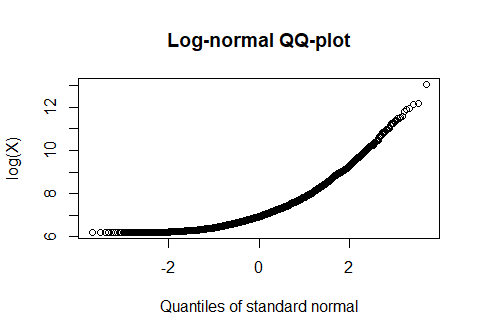# Derivative plot with log X_{n-k,n}
LognormalQQ_der(size)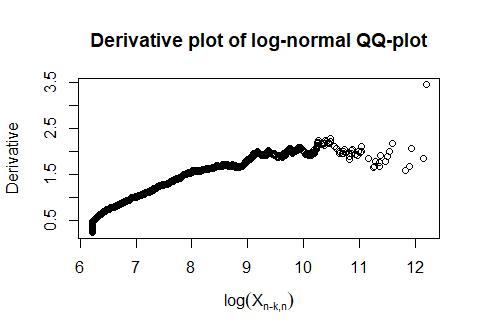# Derivative plot with k
LognormalQQ_der(size, k=TRUE)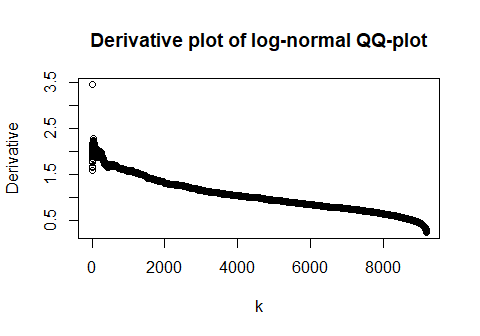## Weibull QQ-plot

Finally, we look at the Weibull QQ-plot (WeibullQQ) and its derivative plot (WeibullQQ_der). The concave shape indicates that the Weibull distribution is not suitable for this data.

# Weibull QQ-plot
WeibullQQ(size)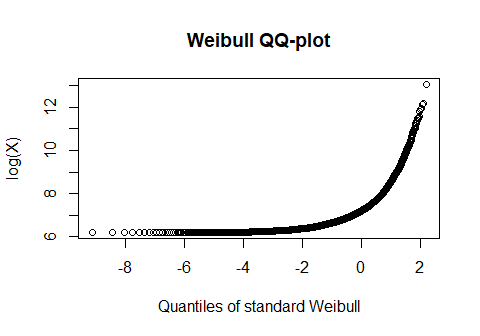# Derivative plot with log X_{n-k,n}
WeibullQQ_der(size)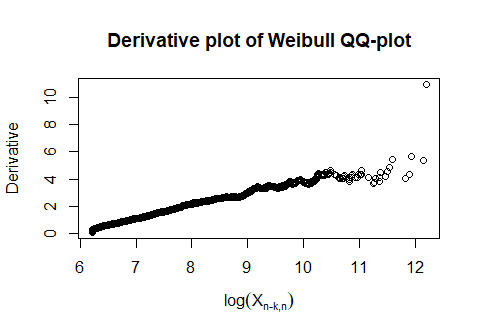# Derivative plot with k
WeibullQQ_der(size, k=TRUE)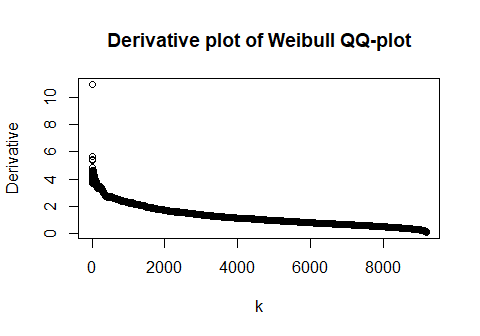# Estimators of the EVI

More details on the estimators described in this section can be found in Section 4.2 in Albrecher et al. (2017).

## Estimators of $$\gamma>0$$

The most famous estimator for the EVI $$\gamma$$ is the Hill estimator which can be obtained by fitting the (strict) Pareto distribution to the relative excesses $$X/X_{n-k,n}$$ using Maximum Likelihood Estimation (MLE). The typical Hill plot can be made using Hill.

The bias of the Hill estimator can be problematic, hence one can consider a bias-reduced estimator which uses a regression-type approach (Hill.2oQV). Another solution is to use the EPD estimator (EPD) which fits the extended Pareto distribution instead of the ordinary Pareto distribution to the relative excesses.

Using these bias-reduced estimators can give an idea about a good choice for $$k$$. Suitable choices of $$k$$ are values where the two plots intersect and which are not too low (otherwise the variance is too high). This yields an estimate for $$\gamma$$ around 0.75 (and $$k$$ around 3500).

# Hill plot
H <- Hill(size, plot=TRUE, col="blue")

# Add EPD estimator
EPD(size, add=TRUE, col="orange", lty=2)

legend("bottomright", c("Hill","EPD"), col=c("blue","orange"), lty=1:2)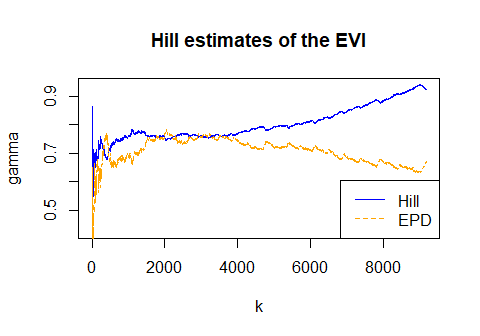## General estimators

The previous estimators can only be used when $$\gamma$$ is strictly positive. Therefore, the generalised QQ-plot was proposed. This QQ-plot is a generalisation of the Pareto QQ-plot and can also have negative, or zero, slopes. The function genQQ needs the Hill estimates as input.

We see that the generalised QQ-plot is strictly increasing which indicates a strictly positive $$\gamma$$.

# Generalised QQ-plot
genQQ(size, gamma=H$gamma)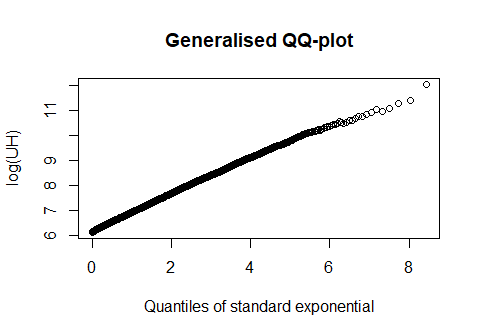The generalised Hill estimator (genHill) is an estimator for the slope of the generalised QQ-plot. Alternatively, one can also consider the moment estimator (Moment) and the Peaks-Over-Threshold estimator (GPDmle) which fits the Generalised Pareto Distribution (GPD) to the excesses $$X-X_{n-k,n}$$ using Maximum Likelihood Estimation (MLE). The Peaks-Over-Threshold estimator can however be very time consuming on large datasets! Therefore, it is omitted in this example. The generalised Hill estimator and the moment estimator indicate that values for $$\gamma$$ around 0.75 are indeed suitable. # Generalised Hill estimator GH <- genHill(size, gamma=H$gamma, plot=TRUE, col="blue", ylim=c(0.5,0.8))

# Moment estimator
M <- Moment(size, add=TRUE, lty=2)

legend("bottomright", c("genHill","Moment"), col=c("blue","black"), lty=1:2)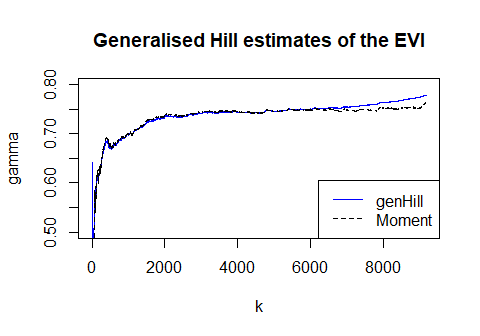# Estimators of quantiles and return periods

The previously discussed estimators can be used to estimate large quantiles or small exceedance probabilities and corresponding high return periods. These estimators are also described in Section 4.2 in Albrecher et al. (2017).

## Estimators of quantiles

We can for example estimate the 99.5% quantile using Hill estimates (Quant) or using generalised Hill estimates (QuantGH), GPD estimates (QuantGPD) and moment estimates (QuantMOM).

All three estimators (we exclude the GPD estimator again) suggest a 99.5% VaR value around 35 000 000 NOK.

p <- 0.005

# Estimates for 99.5% VaR
Quant(size, gamma=H$gamma, p=p, plot=TRUE, col="blue", ylim=c(0,10^5))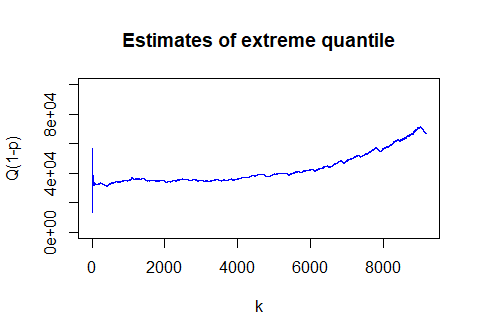# Estimates for 99.5% VaR QuantGH(size, gamma=GH$gamma, p=p, plot=TRUE, col="blue", ylim=c(0,10^5))

QuantMOM(size, gamma=M$gamma, p=p, add=TRUE, lty=2) legend("topright", c("genHill","Moment"), col=c("blue","black"), lty=1:2)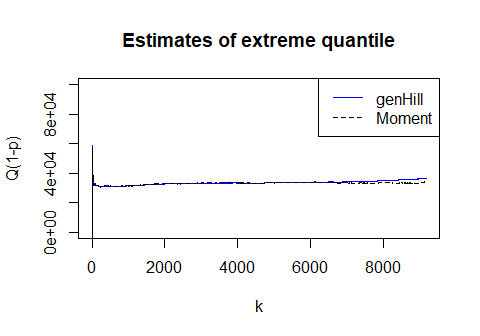## Estimators of return periods Estimating the return period of the value 100 000 000 NOK using the same three estimators (using Return, ReturnGH and ReturnMOM) gives an estimate around 800 (claims). q <- 10^5 # Estimates for return period Return(size, gamma=H$gamma, q=q, plot=TRUE, col="blue", ylim=c(0,1200))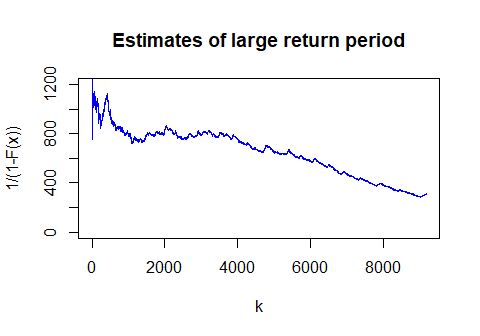# Estimates for return period
ReturnGH(size, gamma=GH$gamma, q=q, plot=TRUE, col="blue", ylim=c(0,1200)) ReturnMOM(size, gamma=M$gamma, q=q, add=TRUE, lty=2)

legend("bottomright", c("genHill","Moment"), col=c("blue","black"), lty=1:2)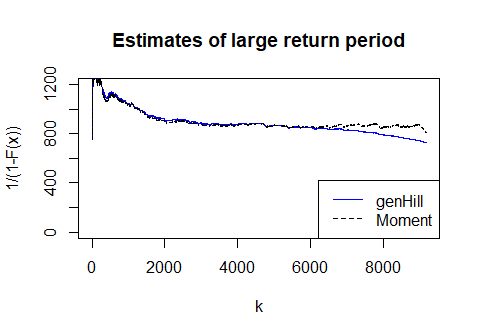# Censored data

## Right censored data

Several EVT estimators for right censored data are included:

• cExpQQ, cLognormalQQ, cParetoQQ, cWeibullQQ: QQ-plots adapted for right censoring.
• cHill, cEPD, cgenHill, cGPD, cMoment: estimators for the EVI.
• cQuant, cQuantGH, cQuantGPD, cQuantMOM: estimators for extreme quantiles.
• cProb, cProbEPD, cProbGH, cProbGPD, cProbMOM: estimators for small exceedence probabilities.

In the example below we plot estimates for the EVI for a simulated sample of right censored data.

# Set seed
set.seed(29072016)

# Pareto random sample
X <- rpareto(500, shape=2)

# Censoring variable
Y  <- rpareto(500, shape=1)

# Observed sample
Z <- pmin(X, Y)

# Censoring indicator
censored <- (X>Y)

# Hill estimates adapted for right censoring
cHill(Z, censored, plot=TRUE)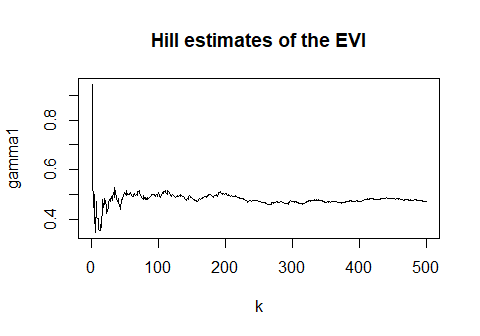## Interval censored data

We also implemented EVT functions for the more general case of interval censored data:

• MeanExcess_TB: mean excess plot adapted for interval censoring (using the Turnbull estimator for the survival function).
• icParetoQQ: Pareto QQ-plot adapted for interval censoring.
• icHill: estimator for (positive) EVI.

In the example below we make the mean excess plot for a simulated sample of right censored data.

# Set seed
set.seed(29072016)

# Pareto random sample
X <- rpareto(500, shape=2)

# Censoring variable
Y  <- rpareto(500, shape=1)

# Observed sample
Z <- pmin(X, Y)

# Censoring indicator
censored <- (X>Y)

# Right boundary
U <- Z
U[censored] <- Inf

# Mean excess plot
MeanExcess_TB(Z, U, censored, k=FALSE)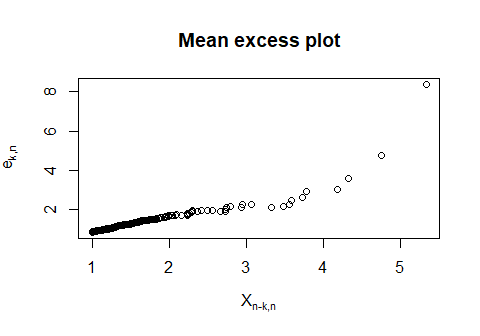# Global fits using splicing

## Fitting a spliced distribution

The previous sections dealt with fitting a suitable distribution for the tail of the data. One usually wants a fit for the whole distribution. We therefore propose the splicing of a Mixed Erlang (ME) distribution for the body and an extreme value distribution, i.e. Pareto or GPD, for the tail. See Section 4.3 in Albrecher et al. (2017) for more details. We consider three possible fitting procedures:

• SpliceFitPareto: fits a splicing model with a ME distribution and Pareto distribution(s) to possibly truncated data.
• SpliceFitGPD: fits a splicing model with a ME distribution and a GPD to possibly lower truncated data. This procedure cannot handle upper truncation.
• SpliceFiticPareto: fits a splicing model with a ME distribution and a Pareto distribution to possibly censored and/or truncated data.

At first, one has to determine (a) suitable splicing point(s). We do this using the mean excess plot. Linear upward pieces indicate that the Pareto distribution is suitable, linear downward pieces suggest a truncated Pareto distribution.

# Mean excess plot
MeanExcess(size)

# Add vertical line at 50% and 99.6% quantiles of the data
abline(v=quantile(size, c(0.5,0.996)), lty=2)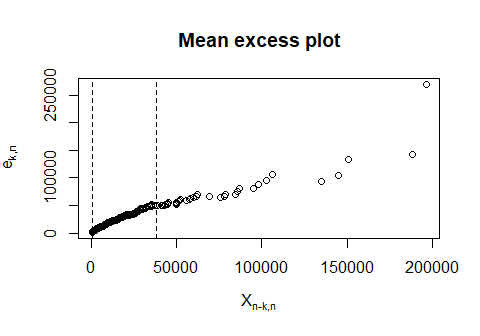For the Norwegian fire insurance data, we choose splicing points at the 50% and 99.6% quantiles. This means that the spliced distribution is a ME distribution between 0 and the first splicing point, a (truncated) Pareto distribution between the first and second splicing point and another Pareto distribution after the second splicing point.

Using SpliceFitPareto we can fit this spliced distribution. This can take some time so we create a SpliceFit object with the obtained parameters to use in the remainder. A summary method is available for these objects which summarises the spliced distribution.

# Splicing of Mixed Erlang (ME) and 2 Pareto pieces
# Use 3 as initial value for M
spliceFit <- SpliceFitPareto(size, const=c(0.5,0.996), M=3)
# Create MEfit object
mefit <- MEfit(p=1, shape=17, theta=44.28, M=1)

# Create EVTfit object
evtfit <- EVTfit(gamma=c(0.80,0.66), endpoint=c(37930,Inf))

# Create SpliceFit object
splicefit <- SpliceFit(const=c(0.5,0.996), trunclower=0, t=c(1020,37930), type=c("ME","TPa","Pa"),
MEfit=mefit, EVTfit=evtfit)

# Show summary
summary(splicefit)
##
## ----------------------------------------
## Summary of splicing fit
## ----------------------------------------
##
## const = (0.5, 0.996)
##
## pi = (0.5, 0.496, 0.004)
##
## t0 = 0
##
## t = (1 020, 37 930)
##
## type = (ME, TPa, Pa)
##
## * * * * * * * * * * * * * * * * * * * *
##
## p = 1
##
## r = 17
##
## theta = 44.28
##
## M = 1
##
## * * * * * * * * * * * * * * * * * * * *
##
## gamma = (0.8, 0.66)
##
## endpoint = (37 930, Inf)

## Checking adequacy of fitted spliced distribution

To see how well the spliced distribution fits the data, we use three tools:

• SpliceECDF: plot of the fitted survival function and the empirical survival function with confidence bounds.
• SplicePP: plot of the fitted survival function vs. the empirical survival function. The plot with minus-log scales is most informative for the tails.
• SpliceQQ: plot of the fitted quantile function vs. the empirical quantile function.

Similar functions for censored data are implemented as well: SpliceTB, SplicePP_TB and SpliceQQ_TB.

We see that the fitted spliced distribution approximates the empirical distribution quite well.

# Points to look at
x <- seq(0, 1*10^5, 10^2)

# Plot of fitted survival function and empirical survival function
SpliceECDF(x, size, splicefit, ylim=c(0,0.1))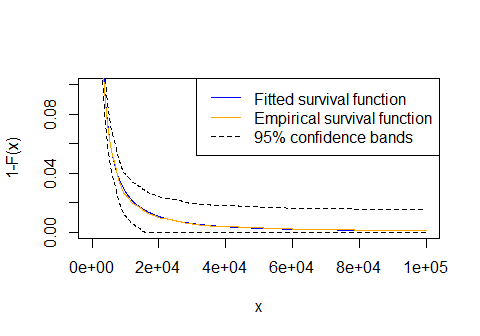# PP-plot with fitted survival function and empirical survival function
SplicePP(size, splicefit)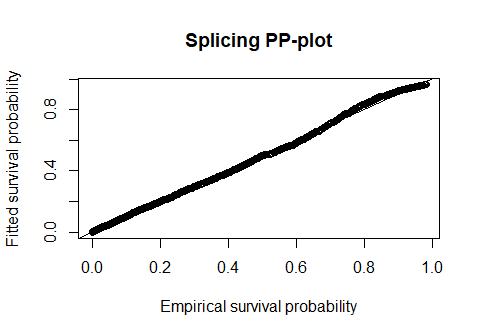# PP-plot with log-scales
SplicePP(size, splicefit, log=TRUE)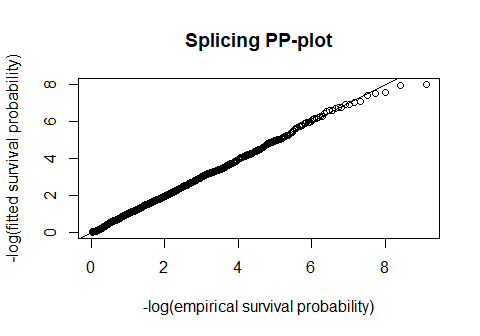# QQ-plot with fitted quantile function
SpliceQQ(size, splicefit)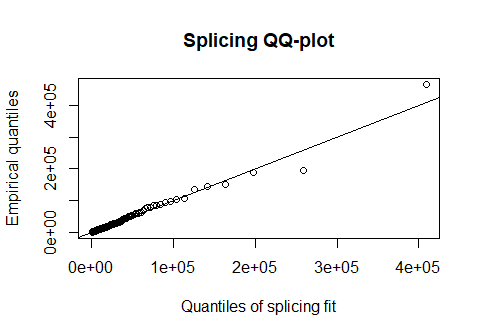# Risk measures

Using the fitted spliced distribution, one can compute estimates for excess-loss premiums, Value-at-Risk (VaR) and Conditional Tail Expectation (CTE). Moreover, data can be simulated from the spliced distribution. See Section 4.6 in Albrecher et al. (2017) for more details.

## Excess-loss premiums

To estimate the premiums of an excess-loss insurance (with retention $$R$$), one can use ExcessSplice. It is also possible to add a limit to the insurance (e.g. $$L=2R$$).

# Sequence of retentions
R <- seq(0, 10^6, 10^2)

# Premiums
e <- ExcessSplice(R, splicefit=splicefit)

# Compute premiums of excess-loss insurance min{(X-M)+,L}
e2 <- ExcessSplice(R, L=2*R, splicefit=splicefit)

# Plot premiums
plot(R, e, type="l", xlab="R", ylab=expression(Pi(R)-Pi(R+L)), ylim=c(0,10^3))
lines(R, e2, lty=2)
legend("topright", c(expression("L"==infinity),expression("L"==2*"R")), lty=1:2, lwd=2)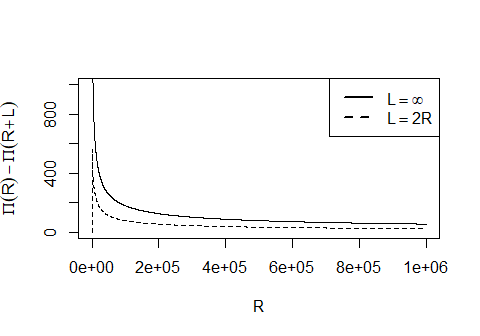## Value-at-Risk

A commonly used risk measure is the Value-at-Risk (VaR). This is nothing more than the quantile $$Q(1-p)$$ of a distribution.

# Take small values for p
p <- seq(0, 0.01, 0.0001)

# VaR
VaR <- VaR(p, splicefit)

# Plot VaR
plot(p, VaR, xlab="p", ylab=expression(VaR[1-p]), type="l")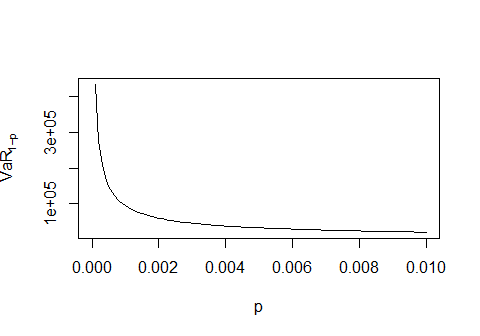## Conditional Tail Expectation

In recent years, the Conditional Tail Expectation (CTE) is gaining importance. It is the conditional expectation of the data above a certain VaR. It is therefore always a larger than the corresponding VaR. When the CDF is continuous, which is the case for the ME-Pa and ME-GPD splicing models, the CTE is equal to the Tail Value-at-Risk (TVaR).

# Small values for p
p <- seq(0.0001, 0.01, 0.0001)

# CTE
cte <- CTE(p, splicefit)

# Plot CTE
plot(p, cte, xlab="p", ylab=expression(CTE[1-p]), type="l")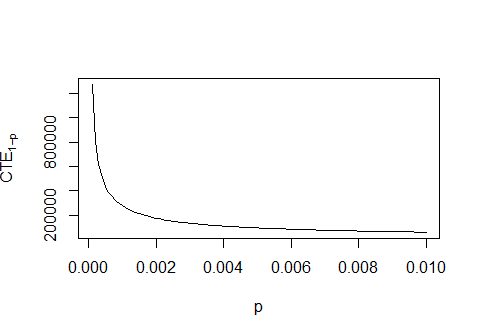## Simulations

Simulation of losses is useful for aggregate loss calculations, e.g. when losses are not independent, and to determine risk measures. The function rSplice simulates data from the fitted spliced distribution.

# Simulate sample of size 1000
X <- rSplice(1000, splicefit)

# Plot simulated sample
plot(X, xlab="Index", ylab="Simulated losses")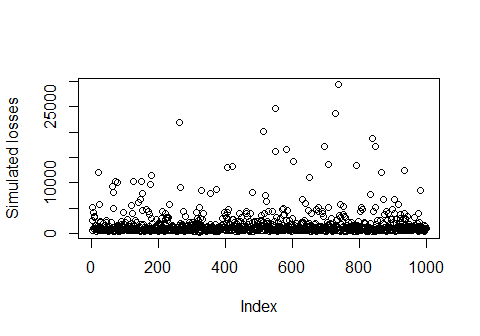# Distributions

Several distributions have been implemented in the package:

• The Burr distribution (type XII): burr.
• The Extended Pareto Distribution (EPD): epd.
• The Fréchet distribution: frechet.
• The Generalised Pareto Distribution (GPD): gpd.
• The Pareto distribution: pareto.
• The spliced distribution consisting of a Mixed Erlang distribution and a Pareto or GP distribution: splice.

Moreover, several upper truncated distributions are included:

• The truncated Burr distribution: tburr.
• The truncated exponential distribution: texp.
• The truncated Fréchet distribution: tfrechet.
• The truncated GPD: tgpd.
• The truncated log-normal distribution: tlnorm.
• The truncated Pareto distribution: tpareto.
• The truncated Weibull distribution: tweibull.

# Approximations of the distribution function

It is often very useful to approximate a distribution using the first moments. See Section 6.2 in Albrecher et al. (2017) for more details on the approximations discussed in this section.

## Classical approximations

Several classical approximations are implemented in the function pClas:

• The normal approximation (method="normal") for the CDF of the r.v. $$X$$ is defined as $F_X(x) \approx \Phi((x-\mu)/\sigma)$ where $$\mu$$ and $$\sigma^2$$ are the mean and variance of $$X$$, respectively, and $$\Phi$$ is the CDF of the standard normal distribution.

• This approximation can be improved when the skewness parameter
$\nu=E((X-\mu)^3/\sigma^3)$ is available. The normal-power approximation (method="normal-power") of the CDF is then given by $F_X(x) \approx \Phi\left(\sqrt{9/\nu^2 + 6z/\nu+1}-3/\nu\right)$ for $$z=(x-\mu)/\sigma\geq 1$$ and $$9/\nu^2 + 6z/\nu+1\geq 0$$.

• The shifted Gamma approximation (method="shifted Gamma") uses the approximation $X \approx \Gamma\left(4/\nu^2, 2/(\nu\times\sigma)\right) + \mu -2\sigma/\nu.$ Here, we need again that $$\nu>0$$.

• The normal approximation to the shifted Gamma distribution (method="shifted Gamma normal") approximates the CDF of $$X$$ as $F_X(x) \approx \Phi\left(\sqrt{16/\nu^2 + 8z/\nu}-\sqrt{16/\nu^2-1}\right)$ for $$z=(x-\mu)/\sigma\geq 1$$. We need again that $$\nu>0$$.

These methods need the mean, variance and possibly the skewness coefficient (all four methods except the normal approximation) as input. Below, the distribution function of the chi-squared distribution is approximated using the four methods.

# Chi-squared sample
X <- rchisq(1000, 2)

x <- seq(0,10,0.01)

# Classical approximations
p1 <- pClas(x, mean(X), var(X))
p2 <- pClas(x, mean(X), var(X), mean((X-mean(X))^3)/sd(X)^3, method="normal-power")
p3 <- pClas(x, mean(X), var(X), mean((X-mean(X))^3)/sd(X)^3, method="shifted Gamma")
p4 <- pClas(x, mean(X), var(X), mean((X-mean(X))^3)/sd(X)^3, method="shifted Gamma normal")

# True probabilities
p <- pchisq(x, 2)

# Plot true and estimated probabilities
plot(x, p, type="l", ylab="F(x)", ylim=c(0,1), col="red")
lines(x, p1, lty=2)
lines(x, p2, lty=3, col="green")
lines(x, p3, lty=4)
lines(x, p4, lty=5, col="blue")

legend("bottomright", c("True CDF", "normal approximation", "normal-power approximation",
"shifted Gamma approximation", "shifted Gamma normal approximation"),
lty=1:5, col=c("red", "black", "green", "black", "blue"), lwd=2)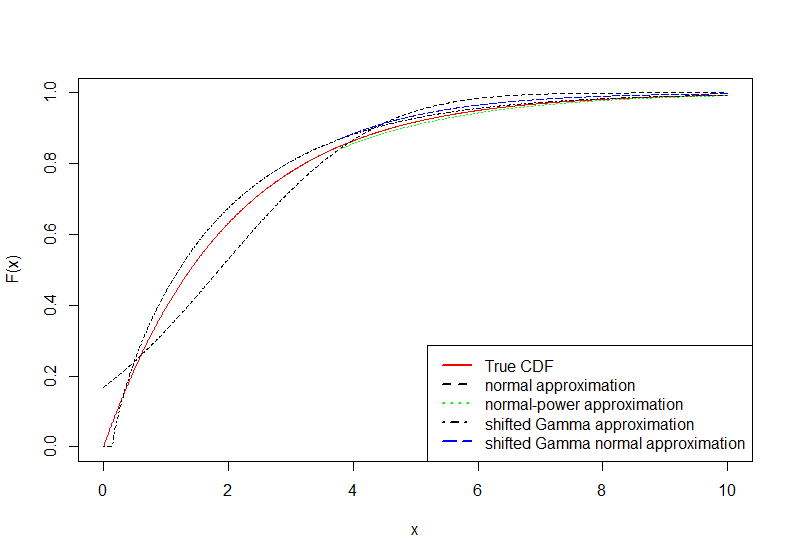## Approximations using orthogonal polynomials

Based on the theory of orthogonal polynomial expansions, the normal approximation can be improved. The Gram-Charlier approximation (pGC) is an improvement of the normal approximation using Hermite polynomials. Another commonly used approximation is the Edgeworth expansion (pEdge).

Denote the standard normal PDF and CDF respectively by $$\phi$$ and $$\Phi$$. Let $$\mu$$ be the first moment, $$\sigma^2=E((X-\mu)^2)$$ the variance, $$\mu_3=E((X-\mu)^3)$$ the third central moment and $$\mu_4=E((X-\mu)^4)$$ the fourth central moment of the random variable $$X$$. The corresponding cumulants are given by $$\kappa_1=\mu$$, $$\kappa_2=\sigma^2$$, $$\kappa_3=\mu_3$$ and $$\kappa_4=\mu_4-3\sigma^4$$. Now consider the random variable $$Z=(X-\mu)/\sigma$$, which has cumulants 0, 1, $$\nu=\kappa_3/\sigma^3$$ and $$k=\kappa_4/\sigma^4=\mu_4/\sigma^4-3$$.

The Gram-Charlier approximation (pGC) for the CDF of $$X$$ is given by $\hat{F}_{GC}(x) = \Phi(z) + \phi(z) (-\nu/6 h_2(z)- k/24h_3(z))$ with $$h_2(z)=z^2-1$$, $$h_3(z)=z^3-3z$$ and $$z=(x-\mu)/\sigma$$.

The Edgeworth expansion (pEdge) for the CDF of $$X$$ is given by $\hat{F}_{E}(x) = \Phi(z) + \phi(z) (-\nu/6 h_2(z)- (3k \times h_3(z)+\nu^2h_5(z))/72)$ with $$h_2(z)=z^2-1$$, $$h_3(z)=z^3-3z$$, $$h_5(z)=z^5-10z^3+15z$$ and $$z=(x-\mu)/\sigma$$.

Both approximations need the first four raw moments as input when raw=TRUE or otherwise the mean, variance, skewness coefficient and kurtosis. Applying the two approximations to the same chi-squared sample as before gives following plot.

x <- seq(0, 10, 0.01)

# Empirical moments
moments = c(mean(X), mean(X^2), mean(X^3), mean(X^4))

# Gram-Charlier approximation
p1 <- pGC(x, moments)

# Edgeworth approximation
p2 <- pEdge(x, moments)

# Normal approximation
p3 <- pClas(x, mean(X), var(X))

# True probabilities
p <- pchisq(x, 2)

# Plot true and estimated probabilities
plot(x, p, type="l", ylab="F(x)", ylim=c(0,1), col="red")
lines(x, p1, lty=2)
lines(x, p2, lty=3)
lines(x, p3, lty=4, col="blue")

legend("bottomright", c("True CDF", "GC approximation",
"Edgeworth approximation", "Normal approximation"),
col=c("red", "black", "black", "blue"), lty=1:4, lwd=2)